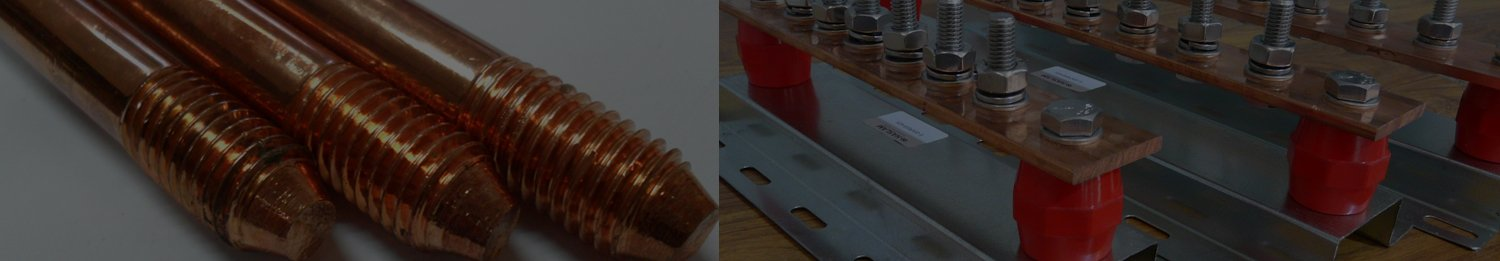# Copper Wire Weight Calculator

Results

To calculate the weight of a copper wire, you'll need to know the dimensions of the wire (typically its diameter or cross-sectional area) and its length. Copper wire weight is determined by its volume and density. Here's the formula:

Weight = Volume × Density

1. Calculate the volume of the wire:

• If you have the diameter (D) of the wire, use the formula for the cross-sectional area (A) of a circle: A = π(D/2)^2
• If you have the cross-sectional area directly, skip this step.
2. Measure the length (L) of the wire in the appropriate units (e.g., meters, feet, inches).

3. Look up the density of copper. The density of copper can vary slightly, but a common value is around 8,960 kg/m³ or 8.96 g/cm³.

4. Plug in the values into the formula:

• If you have the diameter, calculate the volume: Volume = A × L
• If you have the cross-sectional area, Volume = A × L
5. Finally, calculate the weight using the formula: Weight = Volume × Density

Make sure to use consistent units for all measurements. If you're using different units, you might need to convert them to the same unit before doing the calculations.

Here's a summary of the formulas:

If you have the diameter (D) and length (L):

Cross-sectional Area (A) = π(D/2)^2 Volume = A × L Weight = Volume × Density

If you have the cross-sectional area (A) and length (L):

Volume = A × L Weight = Volume × Density

Remember that this calculation assumes a uniform wire with a consistent diameter or cross-sectional area along its length. If the wire isn't uniform, you'll need to break it down into sections with different diameters or areas and calculate their weights separately.